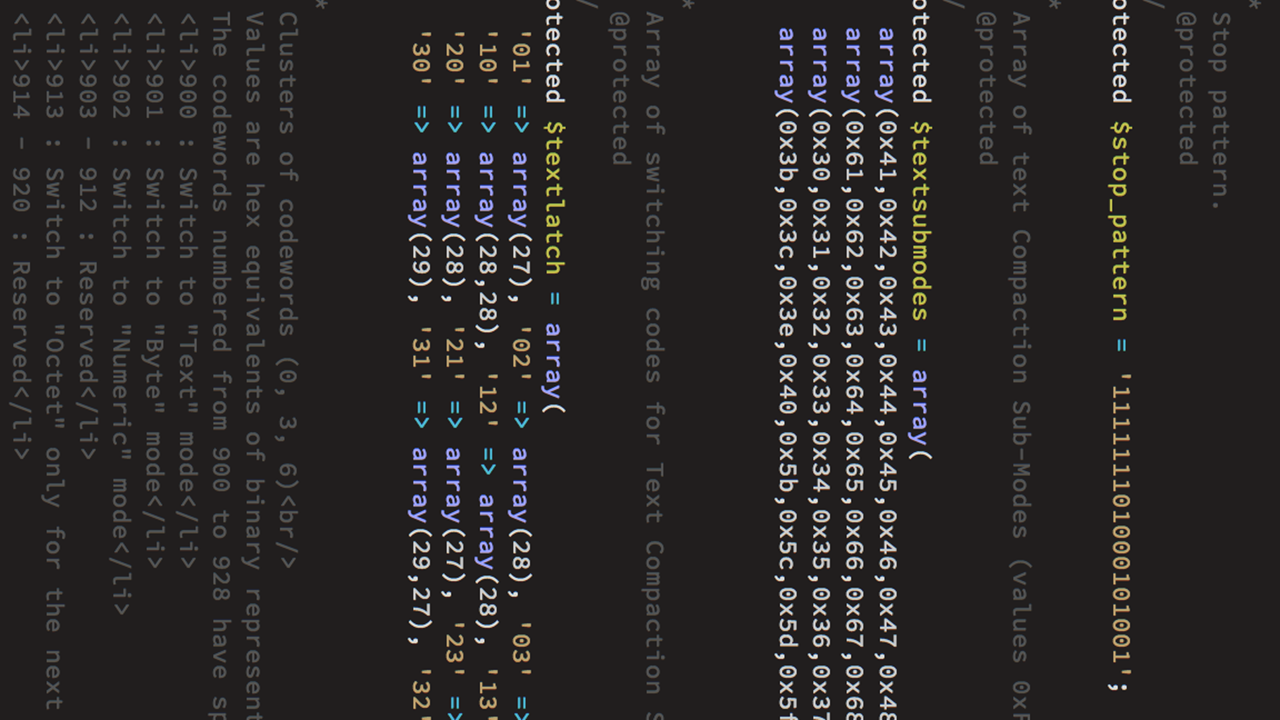# Blogs## Big Data World, Little Solution for Excel

October 26, 2013

One of our awesome customers came to us with an issue: They work with an outside vendor that requires a .xls file with a specific structure. This was a departure from the structure used for many years prior. The XLS data was being exported from a database on a monthly basis, and restructuring the database was not an option. To solve this problem we created an Excel VBA Script to shift the data one column to the right based on specific criteria.

We wanted to share our code as we thought this could be helpful for others. Of course, you’ll need to tweak it based on your data.

```Public intPos As Integer

Function FindString(strCheck As String, strFind As String) As Boolean
intPos = 0
intPos = InStr(strCheck, strFind)
FindString = intPos > 0  '//findstring equals TRUE if intPos greater than zero
End Function

Private Sub MoveTotals()
Dim booWorking As Boolean
Dim rng As Range
Dim itemName As String
Dim Position As Integer
Dim X As Integer
Dim element As Variant
Dim strStates(0 To 29) As String '//array of states to search for

strStates(0) = " IA"
strStates(1) = " MD"
strStates(2) = " NC"
strStates(3) = " GA"
strStates(4) = " FL"
strStates(5) = " MN"
strStates(6) = " DE"
strStates(7) = " MI"
strStates(8) = " ND"
strStates(9) = " TX"
strStates(10) = " AZ"
strStates(11) = " CA"
strStates(12) = " AL"
strStates(13) = " IL"
strStates(14) = " CO"
strStates(15) = " WA"
strStates(16) = " KS"
strStates(17) = " OK"
strStates(18) = " NY"
strStates(19) = " VA"
strStates(20) = " WI"
strStates(21) = " NJ"
strStates(22) = " PA"
strStates(23) = " OH"
strStates(24) = " MO"
strStates(25) = " AR"
strStates(26) = " NV"
strStates(27) = " SD"
strStates(28) = " MS"

Set rng = Cells.SpecialCells(xlCellTypeLastCell)
Set rng = rng.EntireRow.Range("C1") '//select the column

booWorking = True

Do While booWorking '//Stays TRUE while there is data to be read
For Each Cell In rng.Cells
itemName = Cell.Text '//gets the data in each cell and puts it in a  string
X = 0
For Each element In strStates '//compares all of the states against the string

If FindString(LCase(itemName), LCase(element)) Then '//checks for matching characters
Position = InStr(itemName, element)
Position = Position + 2

If Position = Len(itemName) Then '//if it has matching characters and its at the end of the string, move it over
rng.Offset(0, 1).Value = rng.Value
rng.Value = ""
End If
End If
Next element

If (itemName) = "EL CAJON CA" Then
rng.Offset(0, 1).Value = rng.Value
rng.Value = ""
End If

If (itemName) = "OMAHA NE" Then
rng.Offset(0, 1).Value = rng.Value
rng.Value = ""
End If

If (itemName) = "ELKHORN NE" Then
rng.Offset(0, 1).Value = rng.Value
rng.Value = ""
End If

If (itemName) = "NEMAHA NE" Then
rng.Offset(0, 1).Value = rng.Value
rng.Value = ""
End If

If (itemName) = "ST PAUL NE" Then
rng.Offset(0, 1).Value = rng.Value
rng.Value = ""
End If

If rng.Row = 1 Then booWorking = False
If rng.Row > 1 Then Set rng = rng.Offset(-1)

Next Cell
Loop
End Sub
```#### David Birchmier

CEO - At age 15, David & his father built his first computer together. This bonding time also sparked a fire in him to learn more about computers. Fast forward a year or two, David was helping his neighbors & friends with their own tech issues. Even at such a young age, David recognized that one must understand the person & their unique challenge before a real solution can be offered. David holds an AAS & an AA from Indian Hills. He currently resides in Bloomfield and enjoys songwriting and a friendly game of poker.